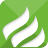# 探索黎曼猜想获证

chf1919@sina.com

Explore the Riemann hypothesis is proven

Chen Hongfa

chf1919@sina.com

Abstract ： According to the number density is normal distribution, the superposition of the probability density using the prime Numbers and sum Numbers for Riemann hypothesis RH proposition proof, and the prime number counting precision formula.

Key words ： the Riemann hypothesis, normal distribution, the probability density, quantum mechanics, prime number counting

1.提出问题

2.研究方法

2.1素数性质

｛自然数N｝=｛0,1｝+｛素数P｝+｛合数C｝，即个数N=2+P+C              （1）

2.2正态分布

P(N)=1-∏(1-Ni-1)=1-e^(∑ln(1-Ni-1))

P(L,T)=1-e^(-L/T)                                                   （2）

2.3素数密度

1-G/N=1-e^(-N/P-N/(N-2-P))

P/(N-2)=0.5±√(0.25-N/(N-2)/ln(N/G))                              （3）

3.命题获证

P/(N-2)=0.5-√(N/(N-2)/ln(N/G)-0.25)i                              （4）

P/(N-2)=e^（2kπ-π/3）i，说明素数分布存在无穷多个并且具有周期性。

4.推理结论

P= N/ ln(N/G)                                                       （5）

N

π（N）

G5

G3

10

4

0.820849986

0.053903576

103

168

2.599643516

0.777677471

105

9592

2.967058336

0.981601093

107

664579

2.918225116

0,999786218

108

5761455

2.897806334

1.002821017

1010

455052511

2.858694683

1.002694038

1013

346065536839

2.821716612

1.001499452

1017

2623557157654233

2.794792213

1.000815672

1027

16352460426841680446427399

2.764668068

1.000296315

P=（N-2）（0.5-√(0.25-N/(N-2)/lnN))

20220412天安和景

0.269443s